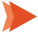Kick Start Your Accounting Journey, We Help You to Start from Scratch

# Net Operating Assets (NOA): Definition, Calculation, and Usage

A company’s net operating assets are its operations-related assets minus its operations-related liabilities. To put it another way, net operational assets are:

+The total assets of a company – All liabilities – All financial assets + All financial liabilities = Net operating assets

The formula for calculating NOA aids firms in determining how much money they have available for operating costs.

Many firms obtain additional funding through financial instruments such as stocks, which must be eliminated from the asset pool in order to obtain a fair picture of net operational assets without the assistance of third parties.

A financial asset generates interest income, and a financial debt causes interest expense. Marketable securities and cash are examples of financial assets, whereas leases and debt are examples of financial liabilities.

Accounts receivable, inventories, and fixed assets are examples of operational assets, while accounts payable and accumulated obligations are examples of operating liabilities.

## NOA Calculation – Operational Methodology

NOA = Operating assets – Operating liabilities = Invested capital

Balance sheets must be restructured such that operating and financing operations can be separated to compute NOA or Invested capital.

Debt and equity investments are examples of financing activities that are not directly tied to the day-to-day operating of the business, such as accounts receivable, inventory, and other accounts that have “interest-bearing” characteristics.

### Operating Assets

The basic formula is as follows –

Operating assets = Total assets – Excess cash and cash equivalents – Financial assets and investment

There should be a difference drawn between liquidity/buffer cash, which is needed for day-to-day operations, and surplus cash, which the organization does not want. Because this distinction is rarely obvious on financial accounts, it must be assumed when computing NOA.

### Explanation of Calculation

• Calculate your entire operating costs. First, take your balance sheet and add up all of your operating assets. An organization’s total operating assets, for example, could be worth \$170,000.
• Calculate the total value of your operational obligations. This amount can also be found on the income statement, and it shows all of the outgoing payments you make to help generate revenue. A company’s entire operational liabilities, for example, could be \$85,500.
• To calculate net operating assets, subtract total operating liabilities from total operating assets using the formula. In the case of the previous example, NOA = (\$170,000) – (\$85,500) = \$84,500. This implies that the firm’s net operating assets are worth \$84,500.

## Example of Net Operating Assets

X has a total asset value of \$5,000,000 and a total liability value of \$2,000,000, resulting in a net asset value of \$3,000,000. X also has \$150,000 in marketable securities and cash, which we deduct from net assets, and \$350,000 in debt, which we re-adjust. The net operational assets, as a result, are \$3,200,000.

## What Is Return on Net Operating Assets?

A firm can generate profits through primarily two sorts of operations. Operating operations through sales are the primary source of revenue. Financial activities are a secondary income source for any company. Investments in bank interest and marketable securities, for example, are types of economic activity.

The goal of return on net operating assets is to separate earnings from operating operations from gains from financial activities. Because financial and investing revenues are not dependent on corporate operations, shareholders are more concerned with returns from the assets used in the firm.

## Net Operating Assets and Net Profit

The ultimate profit statistic that most stakeholders want to see from a company is the net profit. For both investors and managers, it is the bottom line of corporate operations.

Analyzing the operational efficiency of the business is a frequent approach used in net profit and NOA estimates. Both of these metrics evaluate a company’s resources for generating revenue.

To calculate net profit, subtract total revenues from COGS. Then you deduct the business’s operating expenses, such as taxes and interest. The net profit of the company is the result of this calculation.

Net operating assets can be determined in the same method as previously discussed.

Financial assets and liabilities are not included in NOA’s computations. The return generated by operating resources, net of operating liabilities, is the outcome.

As a result, both of these graphs focus on the same metrics. As a result, these two indicators can be combined when measuring a company’s performance.

#### Final Words

The NOA measure distinguishes between a company’s operating and financial income. As a result, it assists a company in determining the primary source of revenue and its efficiency.

Performance analysis indicators such as RNOA can then be used to assess NOA further. In addition, the RNOA ratio is an analytical statistic that connects net profits to net operating assets.

Because liabilities and financial assets are excluded from the computation of NOA, it is unaffected by the business’s leverage level. To put it another way, the metric is not reliant on resources obtained through leverage (debt financing). Likewise, the level of financial responsibilities will have no bearing on it.